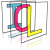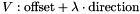Image Component Library (ICL)
icl::geom::ViewRay Struct Reference

This is a view-ray's line equation in parameter form. More...

#include <ViewRay.h>

## Public Types

enum  TriangleIntersection {
noIntersection, foundIntersection, wrongDirection, degenerateTriangle,
rayIsCollinearWithTriangle
}
ray-triangle intersection results More...

## Public Member Functions

ViewRay (const Vec &offset=Vec(), const Vec &direction=Vec(), bool autoNormalizeDirection=false)
Constructor with given offset and direction vector. More...

Vec getIntersection (const PlaneEquation &plane) const
calculates line-plane intersection More...

TriangleIntersection getIntersectionWithTriangle (const geom::Vec &a, const geom::Vec &b, const geom::Vec &c, geom::Vec *intersectionPoint=0, utils::Point32f *parametricCoords=0) const
calculates intersection with given triangle More...

float closestDistanceTo (const Vec &p) const
calculates the closest distance to the given 3D-Point More...

float closestSqrDistanceTo (const Vec &p) const
closest squared distance More...

float closestDistanceTo (const ViewRay &other) const
calculates the closes distance to the given other ViewRay More...

Vec operator() (float lambda) const
evaluates ViewRay' line equation at given lambda More...

## Public Attributes

Vec offset
line offset More...

Vec direction
line direction More...

## Detailed Description

This is a view-ray's line equation in parameter form.

A view-ray is described by the equation## ◆ TriangleIntersection

ray-triangle intersection results

Enumerator
noIntersection
foundIntersection
wrongDirection
degenerateTriangle
rayIsCollinearWithTriangle

## ◆ ViewRay()

 icl::geom::ViewRay::ViewRay ( const Vec & offset = Vec(), const Vec & direction = Vec(), bool autoNormalizeDirection = false )
explicit

Constructor with given offset and direction vector.

## ◆ closestDistanceTo() [1/2]

 float icl::geom::ViewRay::closestDistanceTo ( const Vec & p ) const

calculates the closest distance to the given 3D-Point

for following formula is used:

ViewRay: o + lambda * v;
3D-point: p
distance = sqrt( |p-o|^2 - |(p-o).v|^2 )


## ◆ closestDistanceTo() [2/2]

 float icl::geom::ViewRay::closestDistanceTo ( const ViewRay & other ) const

calculates the closes distance to the given other ViewRay

Here, we use the following formula:

this ViewRay:  o + lambda * v
other ViewRay: k + beta * m
distance = | (k-o).(v x m) |
-----------------
| v x m |


## ◆ closestSqrDistanceTo()

 float icl::geom::ViewRay::closestSqrDistanceTo ( const Vec & p ) const

closest squared distance

## ◆ getIntersection()

 Vec icl::geom::ViewRay::getIntersection ( const PlaneEquation & plane ) const

calculates line-plane intersection

static Camera::getIntersection function

## ◆ getIntersectionWithTriangle()

 TriangleIntersection icl::geom::ViewRay::getIntersectionWithTriangle ( const geom::Vec & a, const geom::Vec & b, const geom::Vec & c, geom::Vec * intersectionPoint = 0, utils::Point32f * parametricCoords = 0 ) const

calculates intersection with given triangle

The actual intersection point in 3D can optionally be stored into a non-null "intersectionPoint" parameter. The parametric triangle coordinats can also optionally be stored in a non-null "parametricCoords" parameter. "parametricCoords" is only written if it is not null and if the returned intersection result is "foundIntersection"

## ◆ operator()()

 Vec icl::geom::ViewRay::operator() ( float lambda ) const

evaluates ViewRay' line equation at given lambda

formula : offset + lambda * direction

## ◆ direction

 Vec icl::geom::ViewRay::direction

line direction

## ◆ offset

 Vec icl::geom::ViewRay::offset

line offset

The documentation for this struct was generated from the following file:
• /Users/alneuman/vm/icl/ICLGeom/src/ICLGeom/ViewRay.h